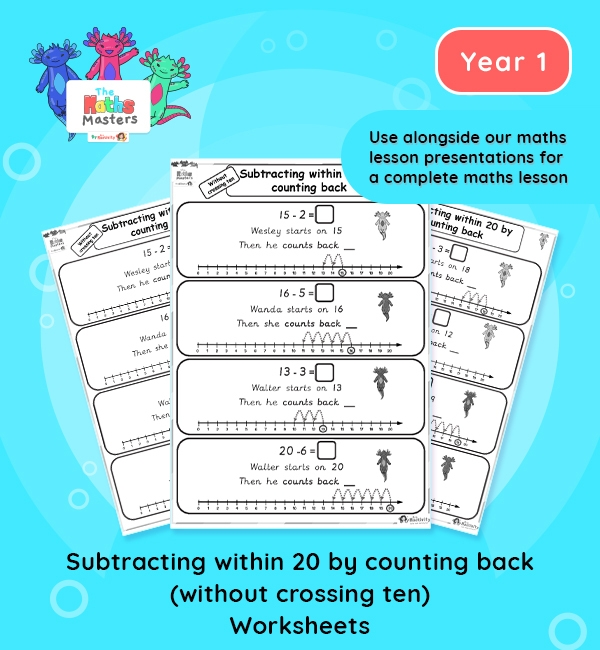# Year 1 | Subtracting Within 20 by Counting Back (Without Crossing 10) Worksheets## Year 1 addition and subtraction worksheets

Aligned with the maths mastery approach, these Year 1 | Subtracting Within 20 by Counting Back (Without Crossing 10) Worksheets are designed to be used alongside our Year 1 | Subtracting Within 20 by Counting Back Lesson Presentation for a complete maths lesson. The maths worksheets requires children to read the simple question and use the number line to count back. The worksheets can also be cut up and used individually in children’s books to demonstrate knowledge and progression, without the need for a whole worksheet.

Topic: Addition and subtraction to 20

This lesson practises subtracting numbers within 20 without crossing ten. It focuses on the method of counting back along a number line.

Small Steps: Subtraction (not crossing ten) – counting back.

NC Links: Read, write and interpret mathematical statements involving addition, subtraction and equals signs. *Add and subtract one-digit and two-digit numbers to 20, including zero. *Solve one step problems that involve addition and subtraction using concrete objects and pictorial representations , and missing number problems.

TAF Statements: Working towards: Add and subtract one digit number explaining their method verbally, in pictures, or using apparatus.

Working At: Recall all the number bonds to and within 10 and use these to reason with and calculate bonds to and within 20, recognising other associated additive relationships.

Greater Depth – Use reasoning about number and relationships to solve more complex problems and explain their thinking.

Ready-to-progress criteria:  1AS-2 Read, write and interpret equations containing addition, subtraction and equals symbols and relate additive expressions and equations to real-life contexts.

Previous experience: Devise and record number stories, using pictures, numbers and symbols.

## Recently Viewed